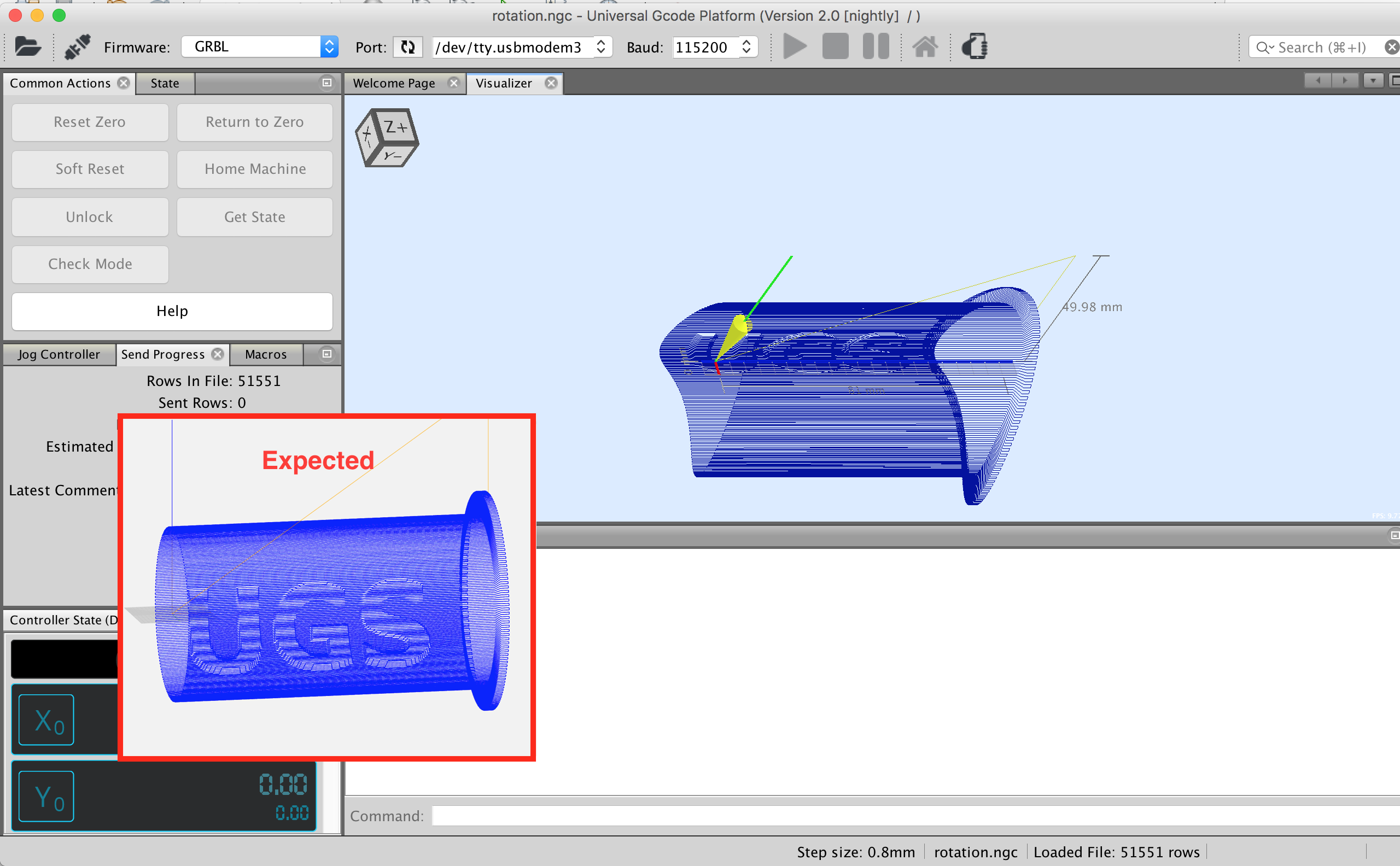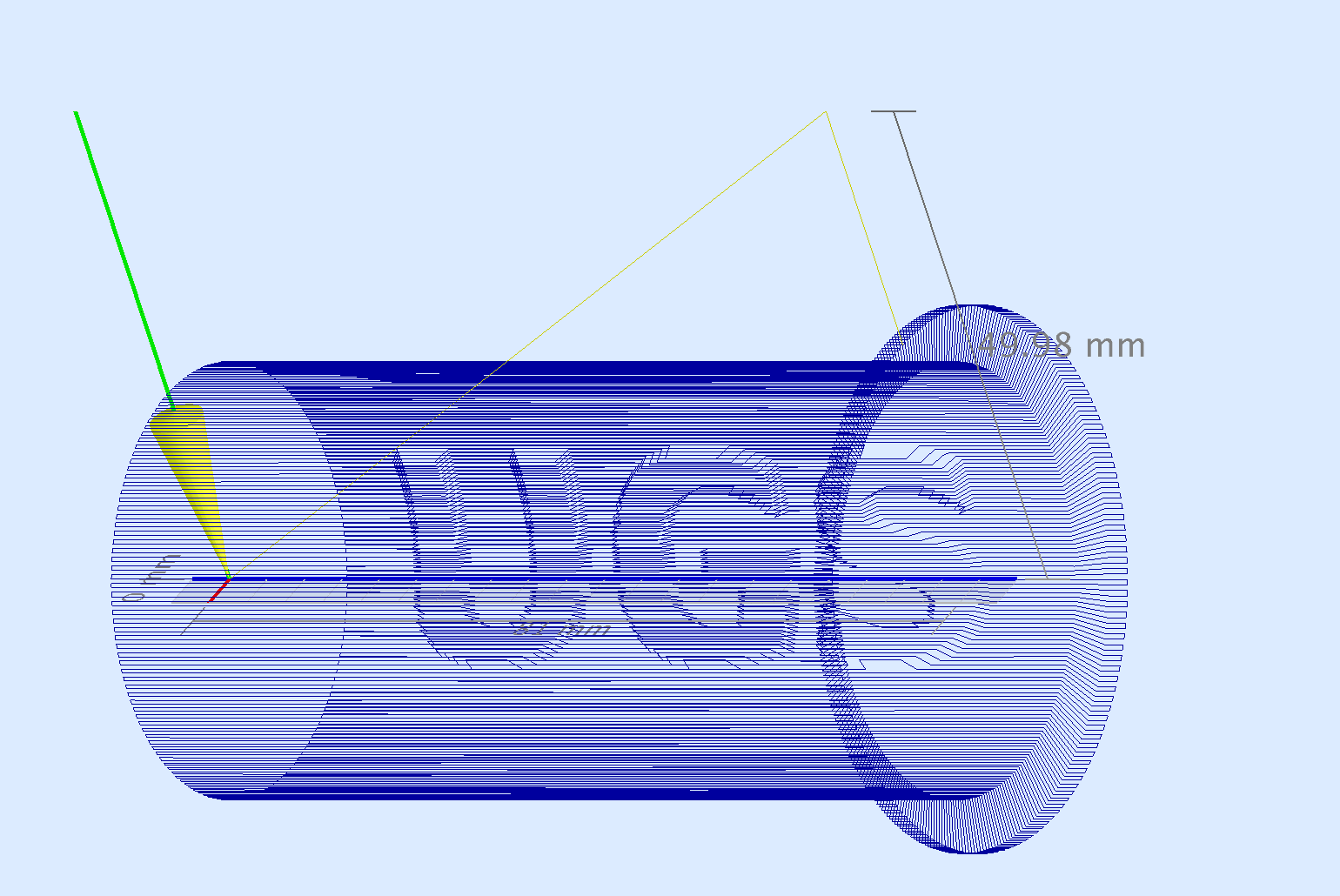开源改变世界!!

# UGS 中的 5 个或更多轴 #1068

4个月前 (01-27) 70次浏览

打开了这个问题 2018 年 6 月 27 日 · 35条评论

# UGS 中的 5 个或更多轴#1068

打开了这个问题 2018 年 6 月 27 日 · 35条评论

## 注释

 嗨，我一直在考虑为 UGS 添加能够使用 3 个以上轴的替代方案。添加辅助轴。我的机器有 5 个轴，但我没有运行它的软件。我想知道这是否可能。感谢收听。
 你用什么固件？ 我最近一直在考虑如何将 ABC 轴添加到可视化工具，但据我所知，GRBL 尚不支持。
评论了 2018 年 6 月 28 日 通过电子邮件
 我拥有一个 GRBL 叉子，它与 RAMPS 1.4 命令结合使用 5 轴 Em 2018 年 6 月 27 日下午 1:11，“Will Winder” escreveu： ……
 谢谢，我大约一周前开始研究这个问题，并且已经取得了一些进展。ABC 轴现在正在被解析并提供给不同的 UGS 小部件。我从可视化工具开始，今天早上我开始处理 XYZ + ABC 位置到 XYZ 图。我的三角函数似乎在某处有问题，但我很高兴有一些与该部分隐约相似的东西。如果有人想仔细检查我的数学，这就是我今天早上做的： ``` private static LineSegment toCartesian(LineSegment p) { Position start = new Position(p.getStart().x, p.getStart().y, p.getStart().z); Position end = new Position(p.getEnd().x, p.getEnd().y, p.getEnd().z); if (p.isRotation()) { double startA = p.getStart().a; double startB = p.getStart().b; double startC = p.getStart().c; double endA = p.getEnd().a; double endB = p.getEnd().b; double endC = p.getEnd().c; // X-Axis rotation // x1 = x0 // y1 = y0cos(u) − z0sin(u) // z1 = y0sin(u) + z0cos(u) if (startA != 0) { double sinA = Math.sin(Math.toRadians(startA)); double cosA = Math.cos(Math.toRadians(startA)); start.y = start.y * cosA - start.z * sinA; start.z = start.y * sinA + start.z * cosA; } if (endA != 0) { double sinA = Math.sin(Math.toRadians(endA)); double cosA = Math.cos(Math.toRadians(endA)); end.y = end.y * cosA - end.z * sinA; end.z = end.y * sinA + end.z * cosA; } // Y-Axis rotation // x2 = x1cos(v) + z1sin(v) // y2 = y1 // z2 = − x1sin(v) + z1cos(v) if (startB != 0) { double sinB = Math.sin(Math.toRadians(startB)); double cosB = Math.cos(Math.toRadians(startB)); start.x = start.x * cosB + start.z * sinB; start.z = -1 * start.x * sinB + start.z * cosB; } if (endB != 0) { double sinB = Math.sin(Math.toRadians(endB)); double cosB = Math.cos(Math.toRadians(endB)); end.x = end.x * cosB + end.z * sinB; end.z = -1 * end.x * sinB + end.z * cosB; } // Z-Axis rotation // x3 = x2cos(w) − y2sin(w) // y3 = x2sin(w) + y2cos(w) // z3 = z2 if (startC != 0) { double sinC = Math.sin(Math.toRadians(startC)); double cosC = Math.cos(Math.toRadians(startC)); start.x = start.x * cosC - start.y * sinC; start.y = start.x * sinC + start.y * cosC; } if (endC != 0) { double sinC = Math.sin(Math.toRadians(endC)); double cosC = Math.cos(Math.toRadians(endC)); end.x = end.x * cosC - end.y * sinC; end.y = end.x * sinC + end.y * cosC; } } LineSegment next = new LineSegment(start, end, p.getLineNumber()); next.setIsArc(p.isArc()); next.setIsFastTraverse(p.isFastTraverse()); next.setIsRotation(p.isFastTraverse()); next.setIsZMovement(p.isZMovement()); next.setSpeed(p.getSpeed()); next.setToolHead(p.getToolhead()); return next; }```
 我快速浏览了你的数学，从我所看到的是正确的，我会停下来冷静地分析它并尝试提出一个解决方案
评论了 2018 年 7 月 3 日

 发现问题了！ 第二行的start.y被修改了……哎呀。这就是我尝试快速制作原型的结果： `````` start.y = start.y * cosA - start.z * sinA; start.z = start.y * sinA + start.z * cosA; `````` 我仍然在某处遗漏了一个减号，文本是倒退的。（编辑：事实证明文本不是倒着的，所有的线都是在工具上绘制的，所以看起来字母在另一边！）@ThiagoAlencar22你有我可以测试的 5 轴 gcode 文件吗？我认为我拥有的代码应该可以工作，但我没有很好的检查方法。
 这真让人兴奋。我也许可以创建一个 5 轴 gcode 文件，但这需要几天时间。
 嗨，早安。这非常令人兴奋。我开始创建一个 gcode 文件，但这也需要几天时间，因为我使用的是 EdgeCam，但我之前从未接触过该软件。
 一个问题。如果我用我发送的 GRBL 在正常程序中加载一个 5 轴的 Gcode。它会运行吗？ 即使没有在 vizualizer 中显示？
 @ThiagoAlencar22是的，它应该可以正常播放。对于 UGS >3 轴支持意味着更新小部件，如 DRO、Visualizer、Jog Controler 等，以显示旋转轴。
 @winder我刚从朋友那里得到一个 Gcode 文件。我会在这里发布并放他的链接
 我只想感谢你们解决这个问题。一开始，一切都是关于 XYZ，而不是轴；’ 已经真正发展到个体电机控制。为了更上一层楼，我们需要能够独立移动每个电机，而不考虑轴。人手大约有 17 个“轴”。为了能够制造出真正令人敬畏的机器人，我们需要无限的轴，并转向“你正在运行多少个步进器？” 模式。我目前有一个 5 电机机械臂，它就放在这里，希望编程能够达到我可以使用 G 代码移动所有机械臂的程度。再次感谢！
 只是一个评论。可能大多数爱好者可以通过添加旋转台来获得 4 轴，旋转台通常垂直于 Y 台安装。MaxNC 4 轴机床就是一个例子。除了上面提到的 GRBL 实现之外，还有 GRBLQ (quatro)，用于 Mega 2560 板，更多引脚。我用过这个并且有效。 我使用 Bobcad（作为爱好者有时可以获得很好的交易）和我扩展到 4 轴的 GRBL g 代码解释器。当然，拥有超过 4 轴将是您程序中受欢迎的补充。感谢分享这么好的程序。TrainDriveShaft_GRBL.txt 许多 CNC 生成程序（爱好者可以负担得起）只是用一个轴代替旋转轴（A – 主）。附上 Y 除了一些舍入外大部分为零的示例。它为 HO 火车制造了一个小型驱动轴，用于将煤车中的电机与发动机耦合。转台安装在工作台左侧。稍后我会看到有关发送所有 4 轴的东西。
 FourAxisSurface_finishPass.txt 这是在复杂表面上进行精加工的 g 代码。在 x、y、z、a 方向移动，其中球头铣刀是垂直的，工作台在 A 方向倾斜以保持刀具垂直于表面，并在 x、y、z 方向移动以进行切割。 UGS 在显示表面方面做得很好，当然在程序运行期间没有倾斜。
评论了 2018 年 8 月 30 日

 出色的。现在快速查看 FourAxisSurface_finishPass.txt 文件，一切看起来都不错。没有错误或其他奇怪的东西不小心留在那里。😁
 谢谢你的文件。只是让他们尝试使用 ABC 分支，而我目前的代码无法解析它们。因此，当我回到这里时，它们将对改进解析器非常有帮助。